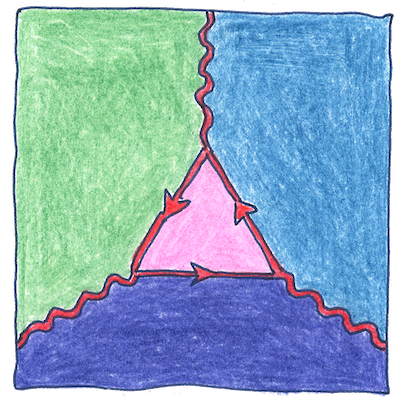# 1671

## The book of science

Tom Sharp

 Jean Richer geodesy

## Gravimetry

• Jean Richer was the first to observe
• that the force of gravity changes
• over Earth’s surface.
• Cassini sent Richer to French Guiana
• to observe Mars during its perihelic opposition.
• There Richer also measured the length
• of the seconds pendulum
• and was surprised to find
• that it was shorter than in Paris.

## Earth’s shape

• Isaac Newton later established
• that gravity between two objects varies
• as the inverse square of the distance between them
• and Newton was the first to conclude
• that Earth’s surface near the equator
• is farther from Earth’s center than in Paris.
• Earth’s shape is an oblate spheroid.
• A cross-section from pole to pole
• is a bumpy ellipse.

## Deviations

• Local topography deviates
• from the earth’s ideal oblate spheroid
• although by very small degrees.
• Local persons sometimes deviate from
• let’s call it the normal, not the ideal,
• thoroughly and dramatically.

The diameter of the earth at its equator is forty-three kilometers greater than the diameter at its poles. This seems like a good distance; however, the top of Mount Everest deviates from the ideal radius of the earth only 0.14%. When calculating the local g, one has to remove the effects of altitude and local topography.

See also in The book of science: